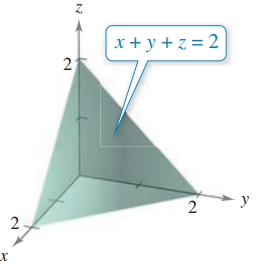Chapter 7.9, Problem 16E### Calculus: An Applied Approach (Min...

10th Edition
Ron Larson
ISBN: 9781305860919

#### Solutions

Chapter
Section### Calculus: An Applied Approach (Min...

10th Edition
Ron Larson
ISBN: 9781305860919
Textbook Problem
1 views

# Finding the Volume of a Solid Region In Exercises 13-20, use a double integral to find the volume of the solid region.To determine

To calculate: The value of the function x+z+y=2 by representing its integral.

Explanation

Given Information:

The provided equation is x+z+y=2.

Formula used:

Standard form of volume integral,

V=z dxdy,z=f(x,y).

Calculation:

Consider the function,

z=f(x,y)f(x,y)=(2xy)at f(x,y)=0; x+y=2

Substitute the given value in primary equation,

V=x=02[y=22x(2xy)dy]dx      V

### Still sussing out bartleby?

Check out a sample textbook solution.

See a sample solution

#### The Solution to Your Study Problems

Bartleby provides explanations to thousands of textbook problems written by our experts, many with advanced degrees!

Get Started

#### Simplify Simplify the rational expression. 21. y2+yy21

Precalculus: Mathematics for Calculus (Standalone Book)

#### In Exercises 516, evaluate the given quantity. log5125

Finite Mathematics and Applied Calculus (MindTap Course List)

#### 2x2 + 8x + 7 = 0

Applied Calculus for the Managerial, Life, and Social Sciences: A Brief Approach

#### 7.Solve

Mathematical Applications for the Management, Life, and Social Sciences